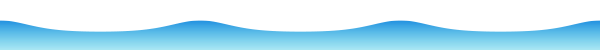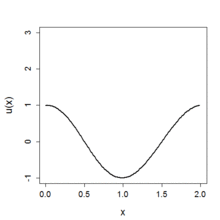# Korteweg–de Vries equationCnoidal wave solution to the Korteweg–de Vries equation, in terms of the square of the Jacobi elliptic function cn (and with value of the parameter m = 0.9).Numerical solution of the KdV equation ut + uux + δ2uxxx = 0 (δ = 0.022) with an initial condition u(x, 0) = cos(πx). Its calculation was done by the Zabusky–Kruskal scheme. The initial cosine wave evolves into a train of solitary-type waves.

In mathematics, the Korteweg–de Vries equation (KdV equation for short) is a mathematical model of waves on shallow water surfaces. It is particularly notable as the prototypical example of an exactly solvable model, that is, a non-linear partial differential equation whose solutions can be exactly and precisely specified. KdV can be solved by means of the inverse scattering transform. The mathematical theory behind the KdV equation is a topic of active research. The KdV equation was first introduced by Boussinesq (1877,footnote on page 360) and rediscovered by Diederik Korteweg and Gustav de Vries (1895).

## Definition

The KdV equation is a nonlinear, dispersive partial differential equation for a functionof two real variables, space x and time t :with ∂x and ∂t denoting partial derivatives with respect to x and t.

The constant 6 in front of the last term is conventional but of no great significance: multiplying t, x, andby constants can be used to make the coefficients of any of the three terms equal to any given non-zero constants.

## Soliton solutions

Consider solutions in which a fixed wave form (given by f(X)) maintains its shape as it travels to the right at phase speed c. Such a solution is given by(x,t) = f(x  ct  a) = f(X). Substituting it into the KdV equation gives the ordinary differential equationor, integrating with respect to X,where A is a constant of integration. Interpreting the independent variable X above as a virtual time variable, this means f satisfies Newton's equation of motion in a cubic potential. If parameters are adjusted so that the potential function V(f) has local maximum at f = 0, there is a solution in which f(X) starts at this point at 'virtual time' ∞, eventually slides down to the local minimum, then back up the other side, reaching an equal height, then reverses direction, ending up at the local maximum again at time ∞. In other words, f(X) approaches 0 as X  ±∞. This is the characteristic shape of the solitary wave solution.

More precisely, the solution iswhere sech stands for the hyperbolic secant and a is an arbitrary constant. This describes a right-moving soliton.

## Integrals of motion

The KdV equation has infinitely many integrals of motion (Miura, Gardner & Kruskal 1968), which do not change with time. They can be given explicitly aswhere the polynomials Pn are defined recursively byThe first few integrals of motion are:

• the mass• the momentum• the energyOnly the odd-numbered terms P(2n+1) result in non-trivial (meaning non-zero) integrals of motion (Dingemans 1997, p. 733).

## Lax pairs

The KdV equationcan be reformulated as the Lax equationwith L a Sturm–Liouville operator:and this accounts for the infinite number of first integrals of the KdV equation (Lax 1968).

## Least action principle

The Korteweg–de Vries equationis the Euler–Lagrange equation of motion derived from the Lagrangian density,withdefined by## Long-time asymptotics

It can be shown that any sufficiently fast decaying smooth solution will eventually split into a finite superposition of solitons travelling to the right plus a decaying dispersive part travelling to the left. This was first observed by Zabusky & Kruskal (1965) and can be rigorously proven using the nonlinear steepest descent analysis for oscillatory Riemann–Hilbert problems.

## History

The history of the KdV equation started with experiments by John Scott Russell in 1834, followed by theoretical investigations by Lord Rayleigh and Joseph Boussinesq around 1870 and, finally, Korteweg and De Vries in 1895.

The KdV equation was not studied much after this until Zabusky & Kruskal (1965), discovered numerically that its solutions seemed to decompose at large times into a collection of "solitons": well separated solitary waves. Moreover, the solitons seems to be almost unaffected in shape by passing through each other (though this could cause a change in their position). They also made the connection to earlier numerical experiments by Fermi, Pasta, Ulam, and Tsingou by showing that the KdV equation was the continuum limit of the FPU system. Development of the analytic solution by means of the inverse scattering transform was done in 1967 by Gardner, Greene, Kruskal and Miura.

The KdV equation is now seen to be closely connected to Huygens' principle.

## Applications and connections

The KdV equation has several connections to physical problems. In addition to being the governing equation of the string in the Fermi–Pasta–Ulam problem in the continuum limit, it approximately describes the evolution of long, one-dimensional waves in many physical settings, including:

The KdV equation can also be solved using the inverse scattering transform such as those applied to the non-linear Schrödinger equation.

### KdV equation and the Gross–Pitaevskii equation

Considering the simplified solutions of the formwe obtain the KdV equation asoror integrating for special case i.e. putting the integration constant to zero:one obtains the generalizedstationary Gross–Pitaevskii equation (GPE)Therefore, for the certain class of solutions of generalized GPE (for the true one-dimensional condensate andwhile using the three dimensional equation in one dimension) two equations are one. While withwith the minus sign and thereal the self-interaction is attractive one should expect the excess in value (bright) soliton solutions.

## Variations

Many different variations of the KdV equations have been studied. Some are listed in the following table.

Name Equation
Korteweg–de Vries (KdV)KdV (cylindrical)KdV (deformed)KdV (generalized)KdV (generalized)KdV (Lax 7th) Darvishi, Kheybari & Khani (2007)KdV (modified)KdV (modified modified)KdV (spherical)KdV (super),KdV (transitional)KdV (variable coefficients)Korteweg–de Vries–Burgers equation1. N.J. Zabusky and M. D. Kruskal, Phy. Rev. Lett., 15, 240 (1965)
2. Darrigol, O. (2005), Worlds of Flow: A History of Hydrodynamics from the Bernoullis to Prandtl, Oxford University Press, p. 84, ISBN 9780198568438
3. See e.g. Newell, Alan C. (1985), Solitons in mathematics and physics, SIAM, ISBN 0-89871-196-7, p. 6. Or Lax (1968), without the factor 6.
4. Alexander F. Vakakis (31 January 2002). Normal Modes and Localization in Nonlinear Systems. Springer. pp. 105–108. ISBN 978-0-7923-7010-9. Retrieved 27 October 2012.
5. See e.g. Grunert & Teschl (2009)
6. Gardner, C.S.; Greene, J.M.; Kruskal, M.D.; Miura, R.M (1967), "Method for solving the Korteweg–de Vries equation", Physical Review Letters, 19 (19): 1095–1097, Bibcode:1967PhRvL..19.1095G, doi:10.1103/PhysRevLett.19.1095.
7. Dauxois, Thierry; Peyrard, Michel (2006), Physics of Solitons, Cambridge University Press, ISBN 0-521-85421-0
8. Fabio A. C. C. Chalub1 and Jorge P. Zubelli, "Huygens’ Principle for Hyperbolic Operators and Integrable Hierarchies"
9. Yuri Yu. Berest and Igor M. Loutsenko, "Huygens’ Principle in Minkowski Spaces and Soliton Solutions of the Korteweg–de Vries Equation", arXiv:solv-int/9704012 DOI 10.1007/s002200050235
10. Shu, Jian-Jun (1987). "The proper analytical solution of the Korteweg-de Vries-Burgers equation". Journal of Physics A: Mathematical and General. 20 (2): 49–56. arXiv:1403.3636. Bibcode:1987JPhA...20L..49J. doi:10.1088/0305-4470/20/2/002.
• Boussinesq, J. (1877), Essai sur la theorie des eaux courantes, Memoires presentes par divers savants ` l’Acad. des Sci. Inst. Nat. France, XXIII, pp. 1–680
• de Jager, E.M. (2006). "On the origin of the Korteweg–de Vries equation". arXiv:math/0602661v1[math.HO].
• Dingemans, M.W. (1997), Water wave propagation over uneven bottoms, Advanced Series on Ocean Engineering, 13, World Scientific, Singapore, ISBN 981-02-0427-2, 2 Parts, 967 pages
• Drazin, P. G. (1983), Solitons, London Mathematical Society Lecture Note Series, 85, Cambridge: Cambridge University Press, pp. viii+136, ISBN 0-521-27422-2, MR 0716135
• Grunert, Katrin; Teschl, Gerald (2009), "Long-Time Asymptotics for the Korteweg-de Vries Equation via Nonlinear Steepest Descent", Math. Phys. Anal. Geom., 12 (3), pp. 287–324, arXiv:0807.5041, Bibcode:2009MPAG...12..287G, doi:10.1007/s11040-009-9062-2
• Kappeler, Thomas; Pöschel, Jürgen (2003), KdV & KAM, Ergebnisse der Mathematik und ihrer Grenzgebiete. 3. Folge. A Series of Modern Surveys in Mathematics [Results in Mathematics and Related Areas. 3rd Series. A Series of Modern Surveys in Mathematics], 45, Berlin, New York: Springer-Verlag, ISBN 978-3-540-02234-3, MR 1997070
• Korteweg, D. J.; de Vries, G. (1895), "On the Change of Form of Long Waves Advancing in a Rectangular Canal, and on a New Type of Long Stationary Waves", Philosophical Magazine, 39 (240): 422–443, doi:10.1080/14786449508620739
• Lax, P. (1968), "Integrals of nonlinear equations of evolution and solitary waves", Comm. Pure Applied Math., 21 (5): 467–490, doi:10.1002/cpa.3160210503
• Miles, John W. (1981), "The Korteweg–De Vries equation: A historical essay", Journal of Fluid Mechanics, 106: 131–147, Bibcode:1981JFM...106..131M, doi:10.1017/S0022112081001559.
• Miura, Robert M.; Gardner, Clifford S.; Kruskal, Martin D. (1968), "Korteweg–de Vries equation and generalizations. II. Existence of conservation laws and constants of motion", J. Mathematical Phys., 9 (8): 1204–1209, Bibcode:1968JMP.....9.1204M, doi:10.1063/1.1664701, MR 0252826
• Takhtadzhyan, L.A. (2001), "K/k055800", in Hazewinkel, Michiel, Encyclopedia of Mathematics, Springer, ISBN 978-1-55608-010-4
• Zabusky, N. J.; Kruskal, M. D. (1965), "Interaction of "Solitons" in a Collisionless Plasma and the Recurrence of Initial States", Phys. Rev. Lett., 15 (6): 240–243, Bibcode:1965PhRvL..15..240Z, doi:10.1103/PhysRevLett.15.240Wikimedia Commons has media related to Korteweg–de Vries equation.